Home > ACC7 > Chapter cc38 > Lesson cc38.1.2 > Problem8-22

8-22.
1. Simplify each expression. Homework Help ✎

1.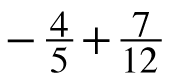2.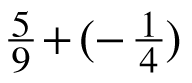3.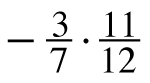4.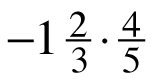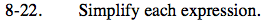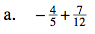Find a common denominator and simplify.

$\left(-\frac{4}{5}\right)\left(\frac{12}{12}\right) + \left(\frac{7}{12}\right)\left(\frac{5}{5}\right)$

$-\frac{13}{60}$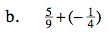Remember that adding a negative number is essentially subtraction.

$\frac{11}{36}$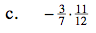Multiply the numerators together and the denominators together.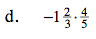Turning the first fraction into an fraction greater than one may prove to be more helpful.